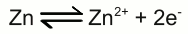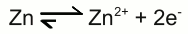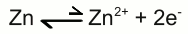# Batteries

## Electrochemical cell

An electrochemical cell is a device capable of either deriving electrical energy from chemical reactions, or facilitating chemical reactions through the introduction of electrical energy. We already recognized the galvanic cell, which provides electric energy from chemical reactions, hence a galvanic cell is a special type of an electrochemical cell. Chemical reactions occurring while electrical energy is introduced will be discussed at the chapter about rechargeable batteries. Like the galvanic cell, an electrochemical cell consists of at least two half cells.

## Equilibrium reactions

The reactions in a half cell of an electrochemical cell are undergoing equilibrium reactions between different oxidation states of the ions respectively atoms. In a half cell, consisting of a zinc electrode and zinc sulfate as electrolyte, the half-reaction is given by:At the state of equilibrium, the concentration of the products doesn't change over time. That doesn't mean, that the reaction stops as soon as the state of equilibrium is reached, but the rate of the forward chemical process (zinc atoms enter the solution as zinc cations) equals those of the reverse reaction (zinc cations are deposited at the surface of the electrode as zinc atoms). On the other hand, if the concentration of the reactant or one of the products changes, the rate of the forward chemical process or the reverse reaction changes, too. For instance, if we would "suck off" the electrons who are created by the forward process, the rate of the forward chemical process would increase, leading to the creation of more electrons:Vice versa, if we would "inject" additional electrons, the rate of the reverse reaction would increase, which consumes electrons by the creation of zinc atoms:It is difficult to "suck off" or "inject" electrons with only one half cell, because the whole half cell would become positively or negatively charged by this procedure. Like described at the chapter about galvanic cells, we need another half cell to do so. If the standard electrode potential of the second half cell is higher, electrons are "sucked off" and anions are transferred into the zinc half cell to balance the charge. For this reason the rate of the forward chemical process is increasing as soon as both half cells are connected through a salt bridge and a wire. On the other hand, if the standard electrode potential of the second half cell is lower, electrons are injected into the zinc electrode and sulfate anions are removed from the zinc electrolyte to balance the charge. Now the rate of the reverse reaction increases.

## Capacity

A galvanic cell converts chemical energy into electrical energy. To calculate the amount of chemical energy, stored in a single galvanic cell, we need to know the number of atoms respectively ions inside of each half cell. Instead of counting the number of atoms, it is much more easy to identify the mass of all atoms inside of an electrolyte respectively electrode. The total mass of the electrode devided through the mass of a single atom gives the number of atoms, the electrode consists of. The mass of a single atom is listed at the periodic table denoted in unified atomic mass units (u). 1u is defined as one twelfth of the rest mass of an unbound atom of carbon-12 in its nuclear and electronic ground state, and has a value of 1.660538782(83)*10-27 kg.
An often used quantity measurement unit in chemistry is mol, which is defined as the amount of substance that contains as many elementary entities (e.g., atoms, molecules, ions, electrons) as there are atoms in 12g of the isotope carbon-12 (12C). Vice versa, the number of atoms contained in 12g of the carbon isotope carbon-12 is given by the Avogadro constant (NA) whose experimentally determined value is 6.02214179(30)*1023 mol-1.
Let's calculate the chemical energy stored in a zinc-copper galvanic cell. During the energy conversion process, the zinc electrode is disintegrated into zinc cations and Cu2+ ions are disposed as elemental copper. Let's say that at the beginning of the process, the mass of the zinc electrode is 50g. The mass of a single zinc atom is 65.409u, so the number of zinc atoms is 0.050kg / (65.409 * 1.661*10-27) = 4.602 * 1023. Each zinc atom looses two electrons while entering the electrolyte as Zn2+ cation, so the number of electrons is twice as much as those of the zinc atoms. The electric energy, transformed by a single electron, is given by the charge of the electron multiplied by the voltage output of the galvanic cell (see equation [3.3] at the chapter voltage). The voltage output is 1.11V and the charge of a single electron -1.602 176 487(40) * 10-19 C, so the total amount of energy is 1.11V * 2 * -1.602 * 10-19 C * 4.602 * 1023 = -163 667Ws = 45.46Wh.
At the other half cell, Cu2+ ions are reduced to copper atoms. So the ratio between zinc atoms and Cu2+ is 1:1, meaning we need the same number of copper ions to dissolve the complete zinc electrode. The copper ions are inside the electrolyte who consists of an aqueous solution of copper(II) sulfate with the chemical formula CuSO4. CuSO4 consists of one copper atom, one sulfur atom, and four oxygen atoms, so the mass of a single CuSO4 compound is: (63.546 + 32.065 + 15.9994 * 4) * 1.661*10-27kg = 2.651*10-25. Multiplied with the number of zinc atoms, we get: 2.651*10-25 * 4.602 * 1023 = 0,122kg. At a temperature of 20°C, maximal 320g CuSO4 can be dissolved in 1L of water, so we need at least: 1L / 320g * 122g = 0.381L of water. Let's say we need the same amount of water for the zinc half-cell, too. So the total weight of the chemicals needed to produce 0.04546kWh is: 50g + 122g + 2 * 381g = 934g. You can use the Java application "Tour de Eco" to see how far you can get with 0.04546kWh.
The amount of energy stored inside a battery is mostly given by ampere-hours (Ah) respectively milliampere-hours (mAh). One ampere hour is the electric charge transferred by a steady current of one ampere for one hour. Hence one ampere-hour is equal to 3600 coulombs (ampere-seconds). To get the accordant energy stored in the battery, the value has to be multiplied with the voltage output of the battery.

## Internal resistance

Like described above, the rate of the forward chemical process or the reverse reaction inside of the half cells depends on the concentration of the reactants respectively products. By altering the concentration of the products, the rate of the forward or reverse reaction is altered, too. The voltage potential of a half cell, listed at the table of the standard electrode potentials, assumes that the state of equilibrium is reached. As soon as the terminals of the zinc-copper battery, given at the example above, are connected to a load, a current flows and the number of electrons at the zinc anode is decreasing with the accordant rate. The lower the resistance of the load, the higher the number of electrons being "sucked off" at a given time interval. At the copper electrode, Cu2+ ions leave the electrolyte by gaining the electrons of the zinc electrode. To keep the current flowing, the accordant number of Cu2+ ions has to be near the electrode to be able to leave the electrolyte. If the concentration of Cu2+ ions around the copper cathode is decreasing, the rate of the forward reaction is increasing, hence at a given time interval, a fewer number of Cu2+ ions is reduced to copper atoms:As a consequence of the increasing reaction rate of the forward process, the voltage output of the battery decreases! The motion speed of the Cu2+ ions affects the reaction rate at the copper electrode:Zinc copper battery:
When a load is connected to the terminals of the battery, the chemical reactions at the two half cells lead to inhomogeneous arrangements of the ions around the electrodes. The concentration of Zn2+ around the anode increases, those of Cu2+ ions around the cathode decreases.

As soon as a load is connected to a battery, the voltage output is lower than the no-load voltage. The difference is the voltage drop caused by the internal resistance of the battery.